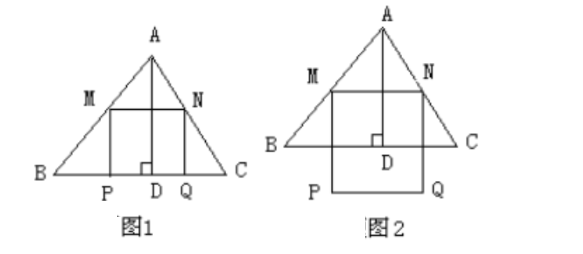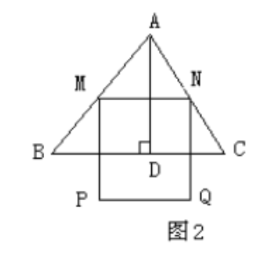$M N // B C$, 以 $\mathrm{MN}$ 为边向下作正方形 $M P Q N$, 设其边长为 $\mathrm{x}$, 正方形 $M P Q N$ 与 $\triangle A B C$ 公共部分的(1) $\triangle A B C$ 中边 $\mathrm{BC}$ 上高 $\mathrm{AD}=$
(2) 当 $x=$ 时,PQ 恰好落在边 $\mathrm{BC}$ 上（如图 1)。
(3) 当 $\mathrm{PQ}$ 在 $\triangle A B C$ 外部时 (如图 2), 求 $\mathrm{y}$ 关于 $\mathrm{x}$ 的函数关系式 (注名 $\mathrm{x}$ 的取值范围), 并求出 $\mathrm{x}$ 为何值时 $\mathrm{y}$ 最大, 最大值是多少?

(1) $A D=4$;
(2) $x=2.4$;(3) 设 $B C$ 分别交 MP、NQ于 $E 、 F$, 则四边形 MEFN 为矩形。

\begin{aligned} & \because M N // B C, \\ & \therefore \triangle A M N \sim \triangle A B C \\ & \therefore \frac{M N}{B C}=\frac{A G}{A D}, \text { 即 } \frac{x}{6}=\frac{4-h}{4} \\ & \therefore h=-\frac{2}{3} x+4 . \\ & \therefore y=M N \cdot N F=x\left(-\frac{2}{3} x+4\right) \\ & =-\frac{2}{3} x^2+4 x(2.4 < x < 6) \end{aligned}